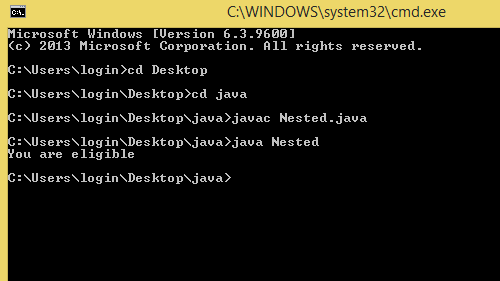×

### Html & Html5

Introduction to JAVA

JAVA

Html Form

Html5 basics

Html5 Grid

Html5 Graphics

Html5 Media

Html5 APIs

### JAVA

Intro of JAVA

Learn Java

Decision making or control statements are used to control the flow of execution of program.There are different kind of control statements as follows:

1. If-statement
2. Loop Statement
3. Jump Statement

## IF Statement

There are various kind of if statement:

1. if
2. if-else
3. if-else-if
4. nested if

Let us explain each statement-

### If statement

If statement decide to execute the block of statement or not.It execute the statement only if condition is TRUE.

Syntax:-

if(condition){

// statement to be executed

}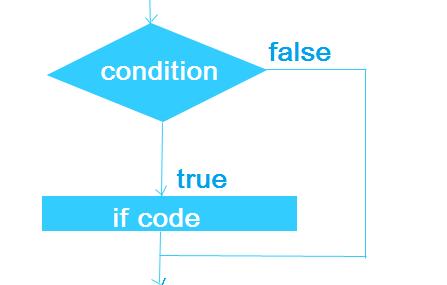Example:

Class Abc{

public static void main(String args[]){

int i=3;

if(i<=6)

{

System.out.println(i);}

}

}

Output: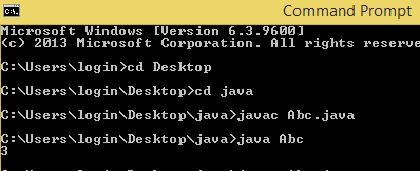### If-else statement

If-else statement execute if block if condition is true otherwise it execute else block of statement.

Syntax:-

if(condition){

statement 1//statement 1 is executed if condition is true

}

else{

statement 2//statement 2 is executed if condition is false

}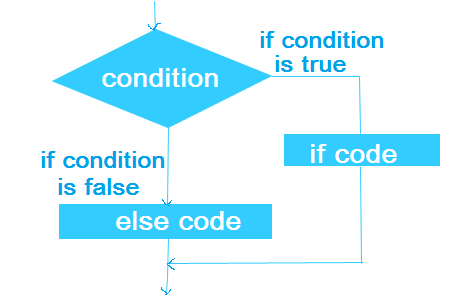Example:

class Ifelse{

public static void main(String args[]){

int number=10;

if(number<=5)

{

System.out.println("if true"+number);}

else{

System.out.println("if false"+number);}

}

Output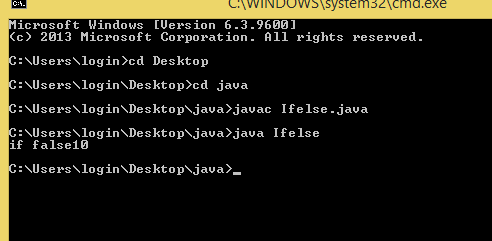### If-else-If statement

If-else-If statement execute one statement from multiple statements. if no statement matches condition then it will execute final statement. execute else

Syntax:-

if(condition1){

Statement1;// executes if condition 1 is true

}

else if(condition 2){

Statement 2;// executes if condition 2 is true

}

else if(condition 3){

Statement 3;// executes if condition 3 is true

}

else

Statement3;//executes if no above condition is true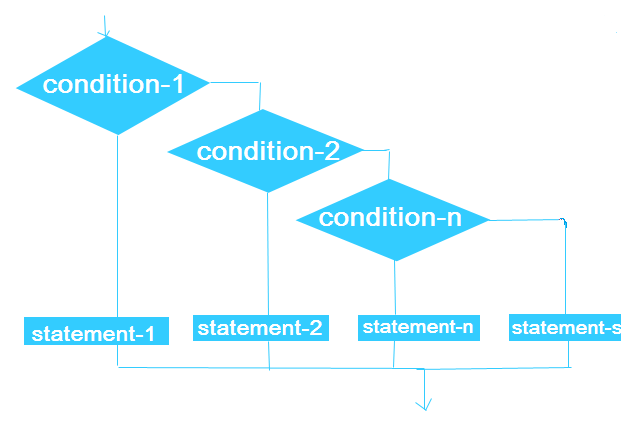Example:

public class IfElseIf {

public static void main(String[] args) {

int marks=65;

if(marks<50){

System.out.println("fail");}

else if(marks>=50 && marks<60){

else if(marks>=60 && marks<70){

}

else if(marks>=70 && marks<80){

}

else if(marks>=80 && marks<90){

}else if(marks>=90 && marks<100){

}else{

System.out.println("Invalid!");

}

}

};

Output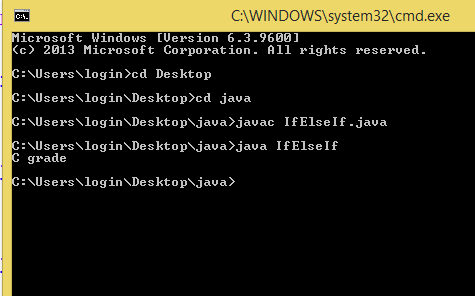### Nested-If statement

Nested-If statement means if statement is enclose within another if statement.

Syntax:-

if(condition1){

//code1 to be executed

{

if(condition2)

//code 2to be executed}

}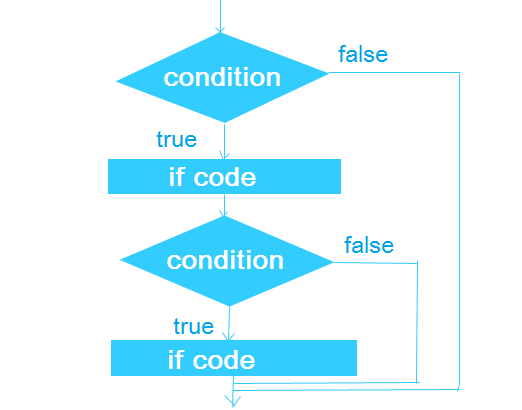Example:

public class Nested {

public static void main(String[] args) {

//Creating two variables for age and weight

int age=20;

int weight=80;

//applying condition on age and weight

if(age>=18){

if(weight>50){

System.out.println("You are eligible");

}

}

}

};

Output